# RD Sharma Solutions For Class 12 Maths Exercise 19.30 Chapter 19 Indefinite Integrals

RD Sharma Solutions for Class 12 Maths Exercise 19.30 Chapter 19 Indefinite Integrals is provided here. RD Sharma Solutions for Class 12 PDF can be downloaded effortlessly by students to score well in the exam. The faculty at BYJU’S prepare solutions for the exercise wise problems after conducting wide research on each concept.

Students can solve problems from the RD Sharma textbook by referring to the solutions to self analyse their weaknesses. This exercise deals with the integration of rational algebraic functions by using partial fractions. The PDF of RD Sharma Solutions for Class 12 Maths Chapter 19 Exercise 19.30 is provided here. The important topics of this exercise are listed below.

• When the denominator is expressible as a product of distinct linear factors
• When denominator contains some repeating linear factors
• The denominator contains irreducible quadratic factors

## Download the PDF of RD Sharma Solutions For Class 12 Chapter 19 – Indefinite Integrals Exercise 19.30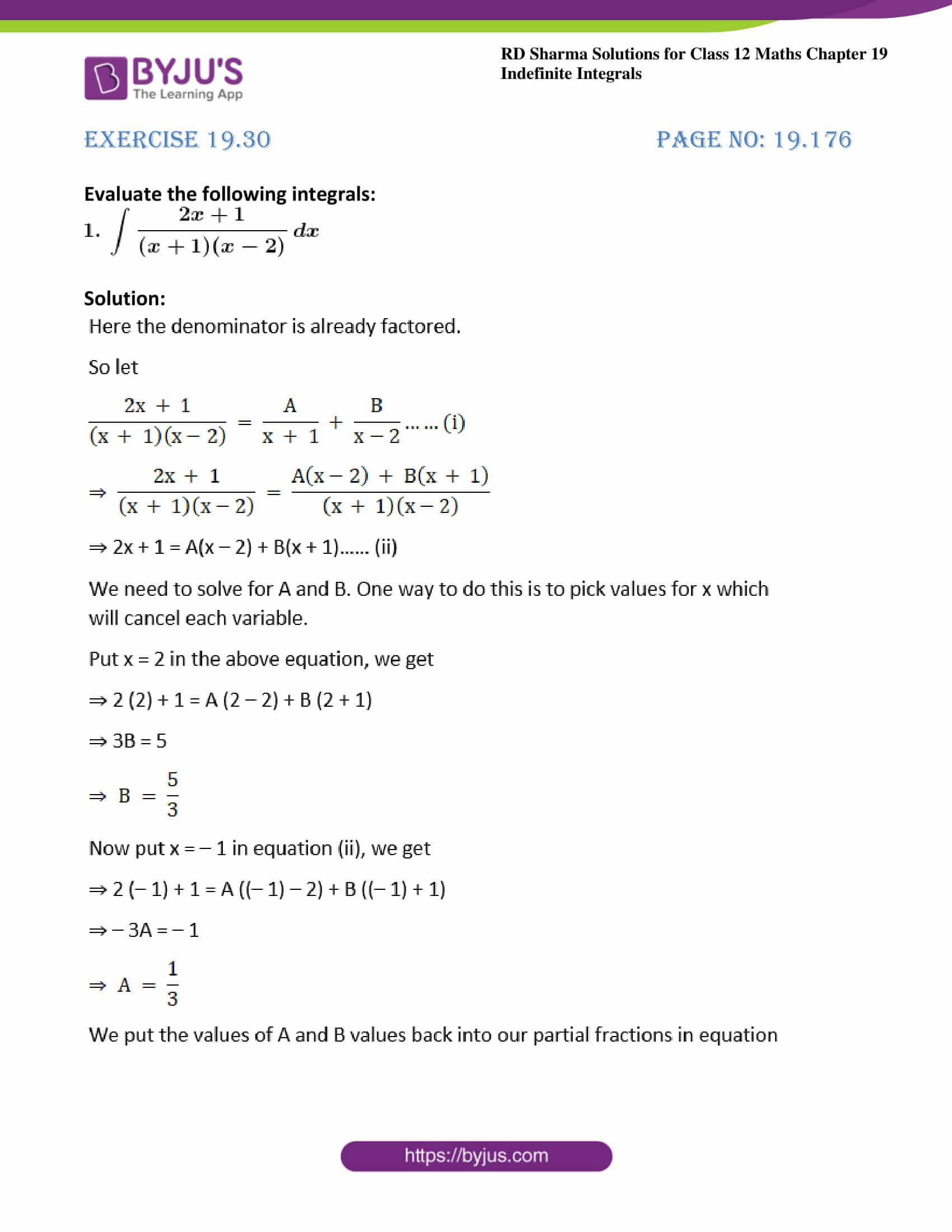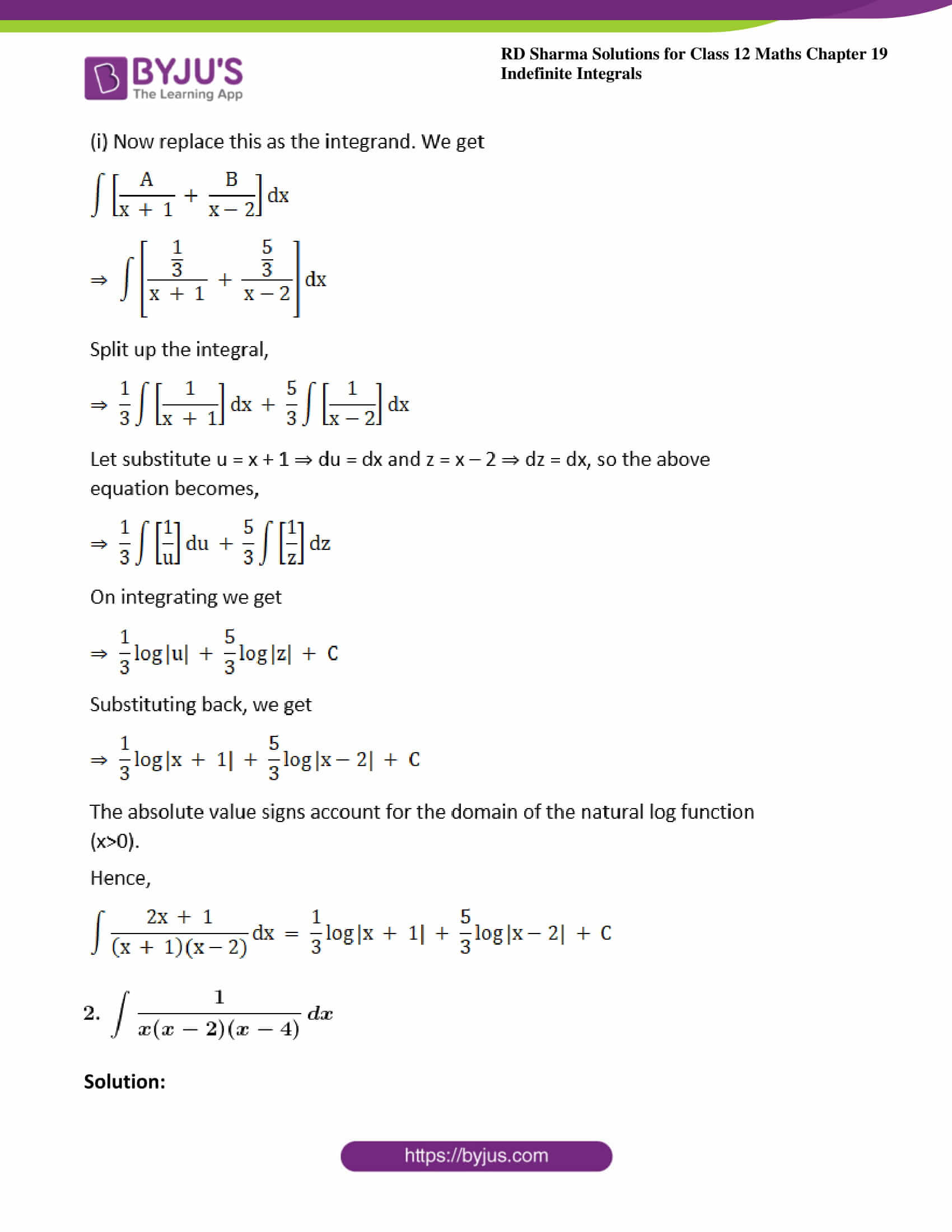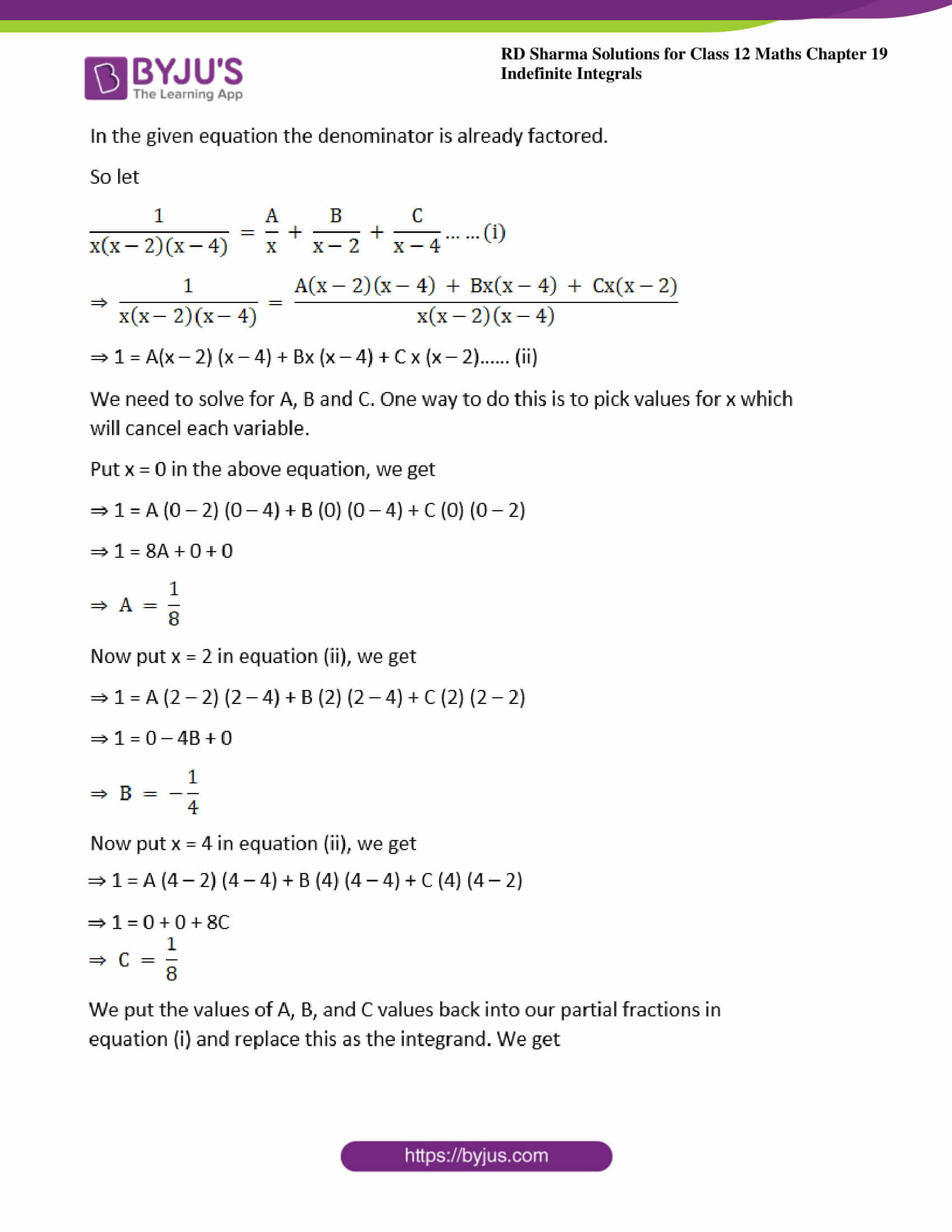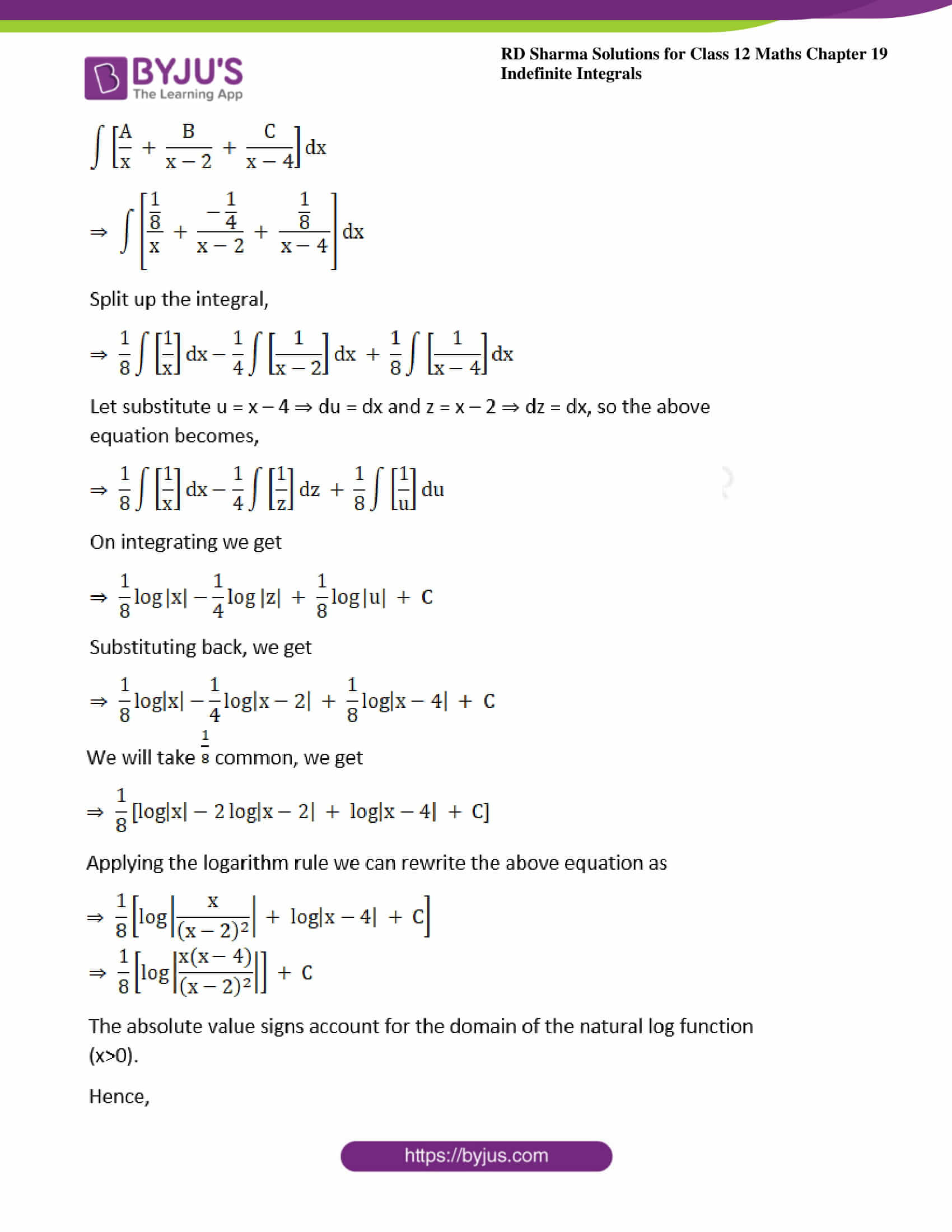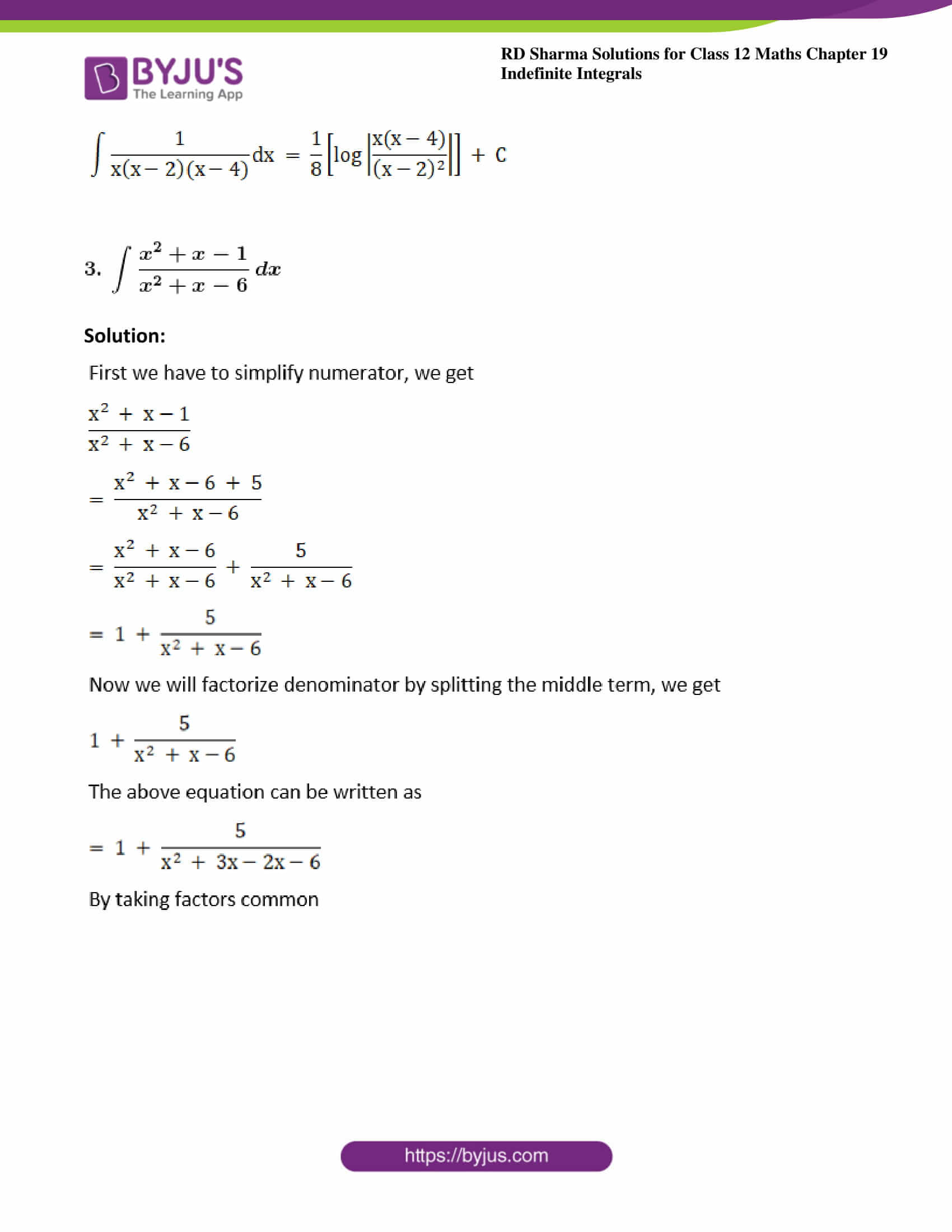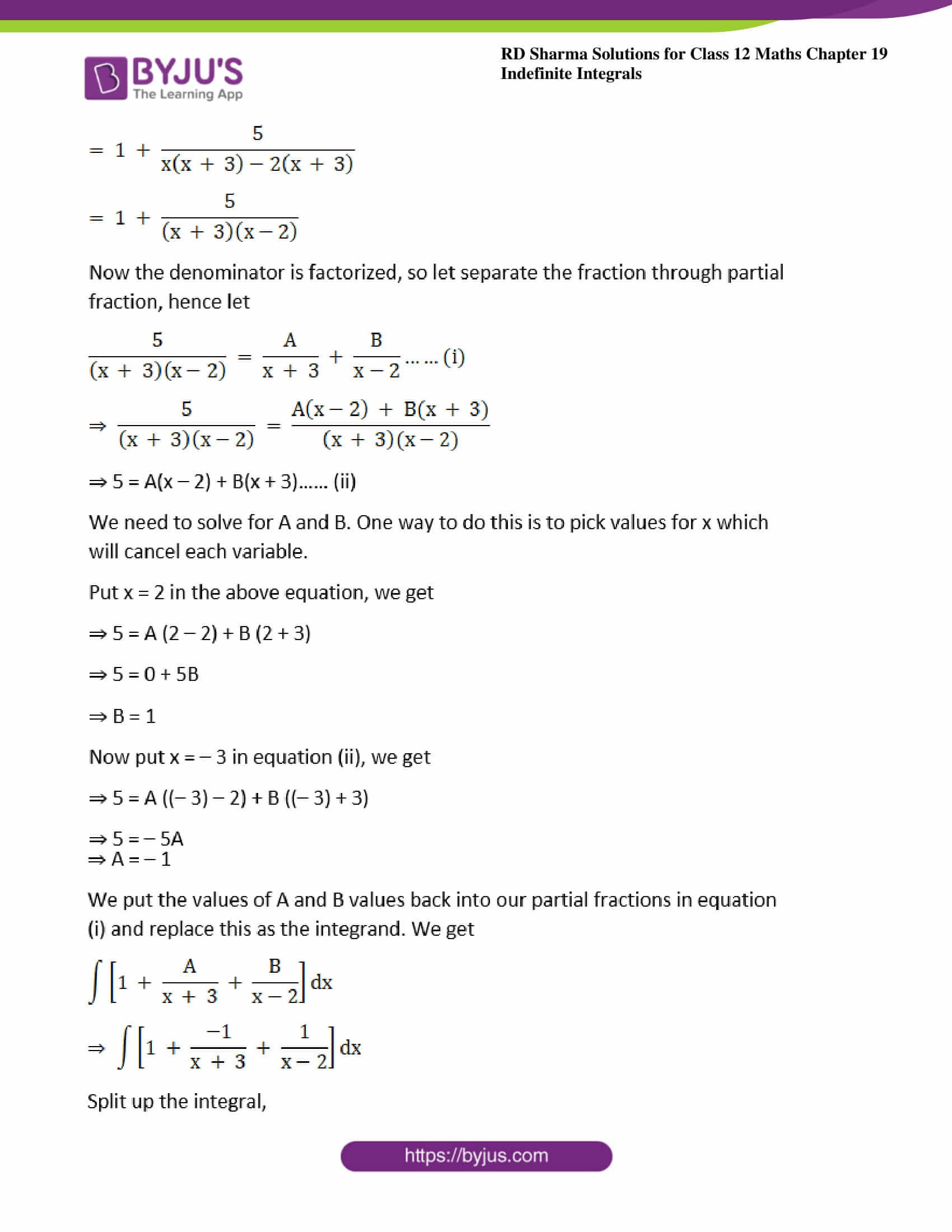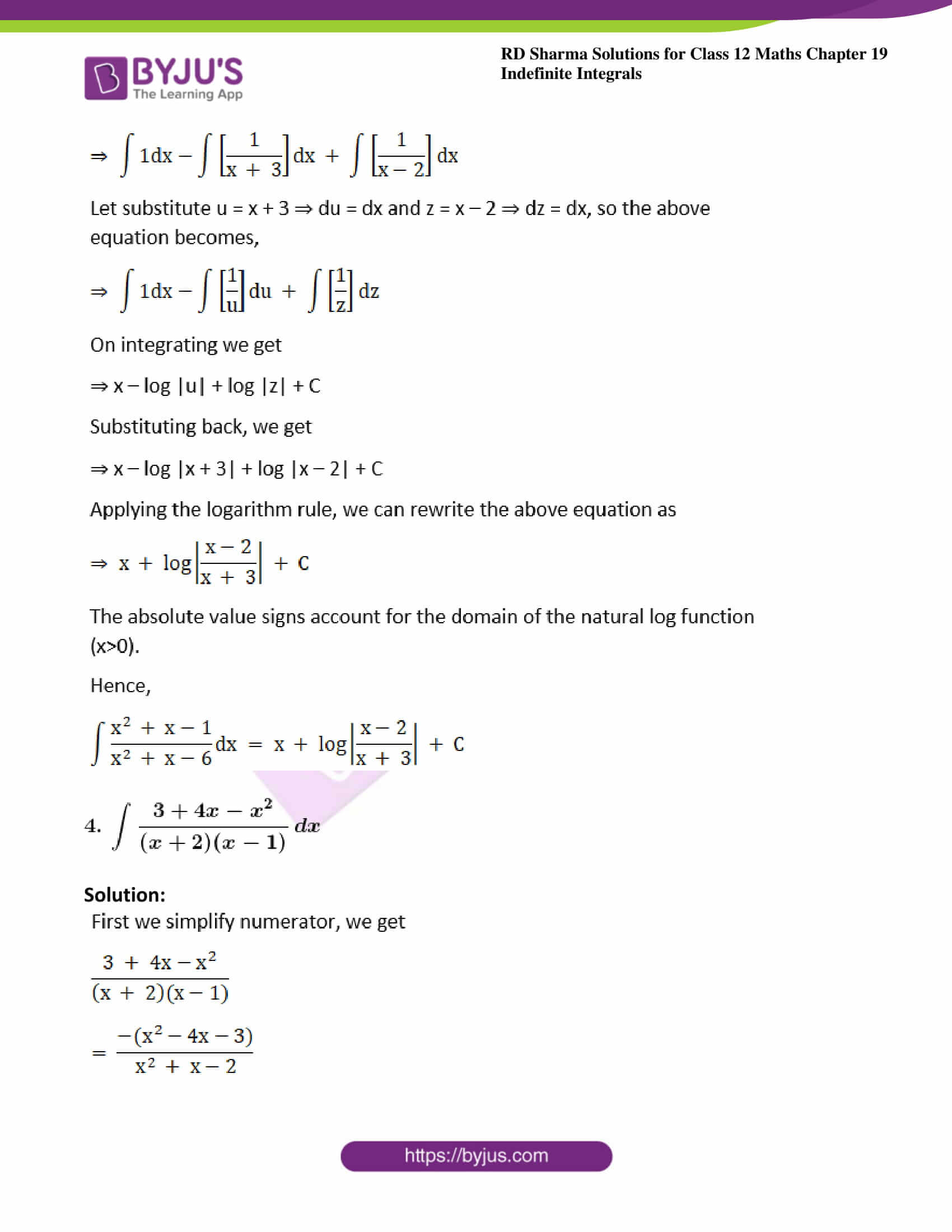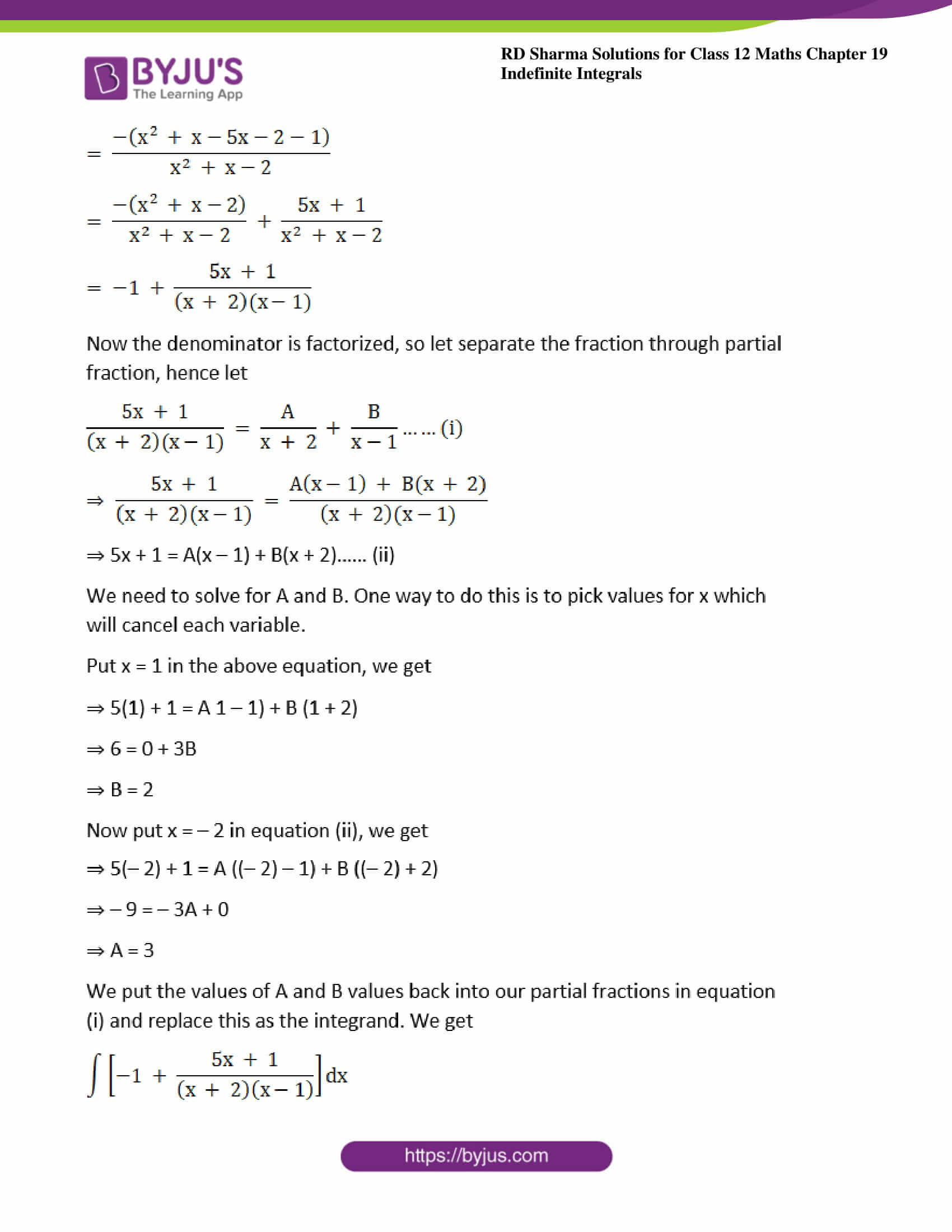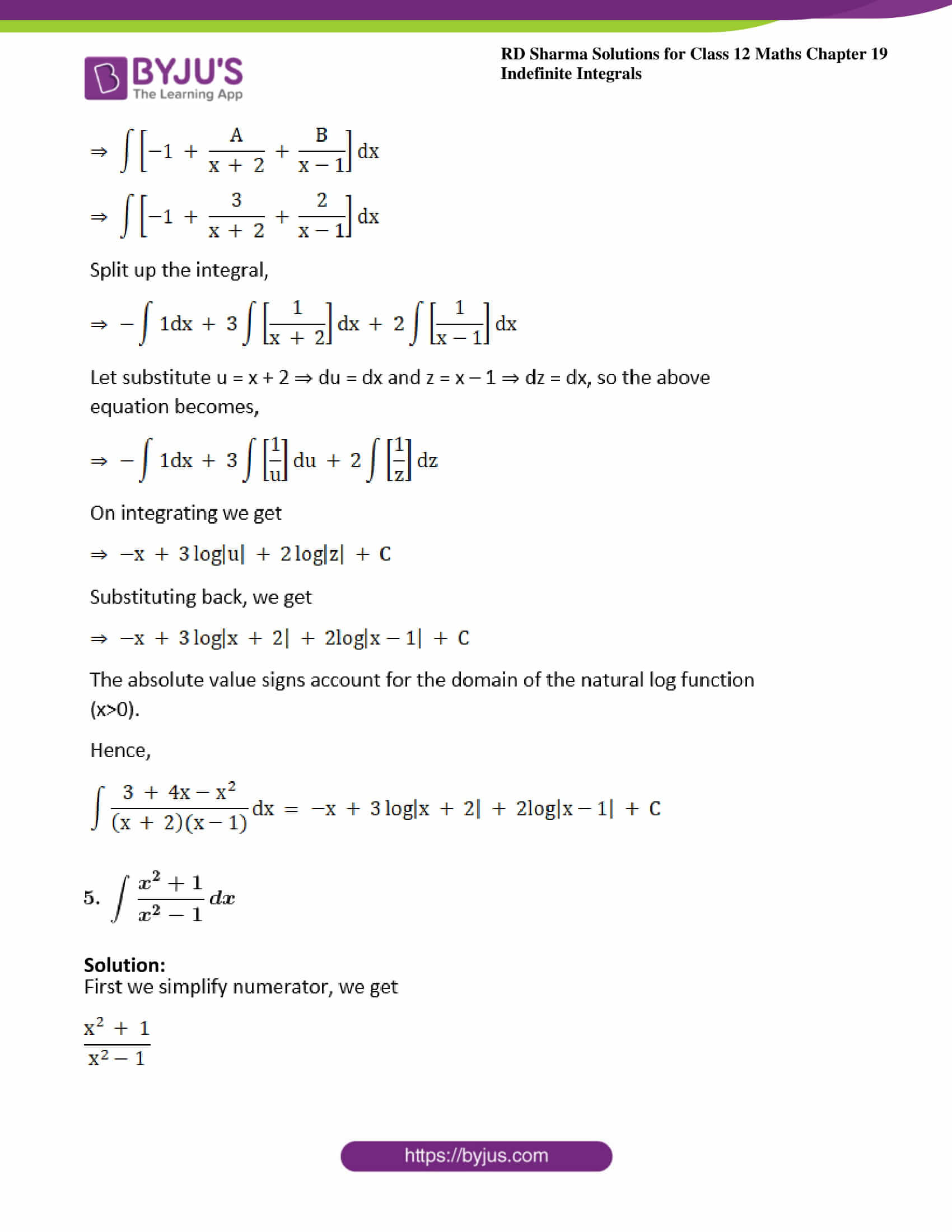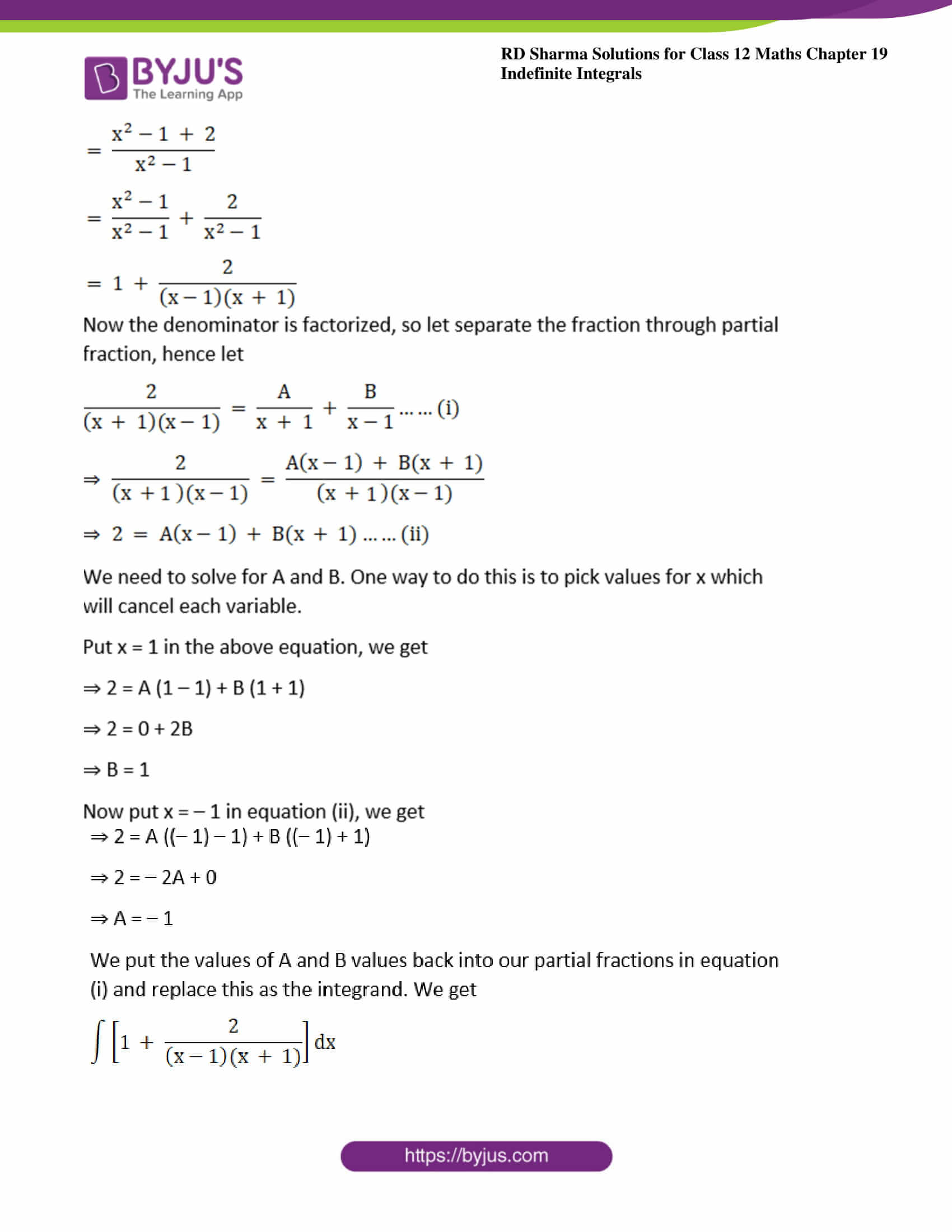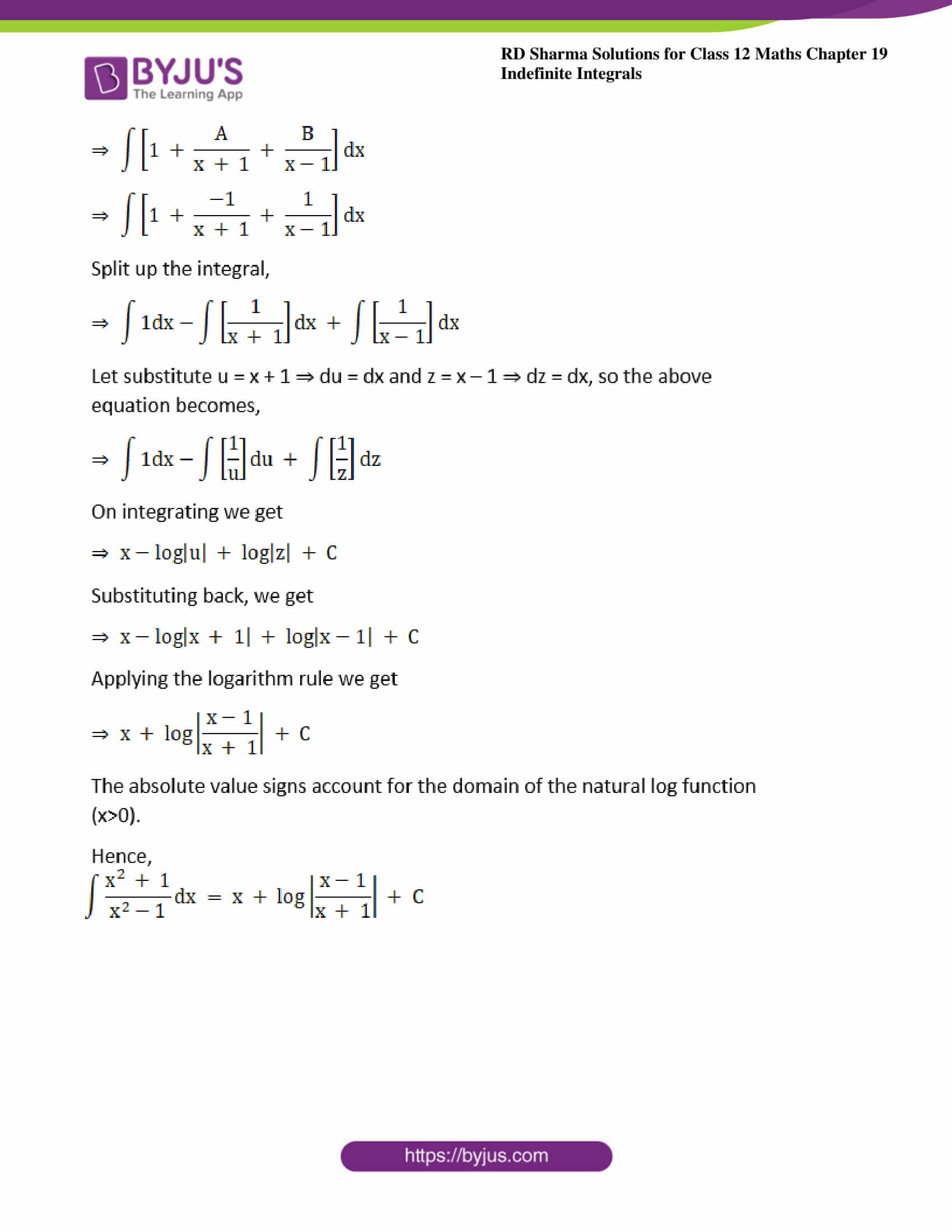### Exercise 19.30 Page No: 19.176

Evaluate the following integrals: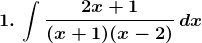Solution: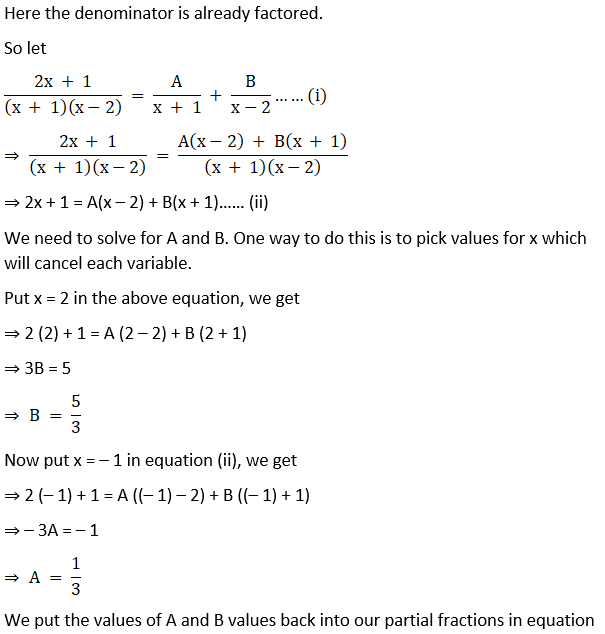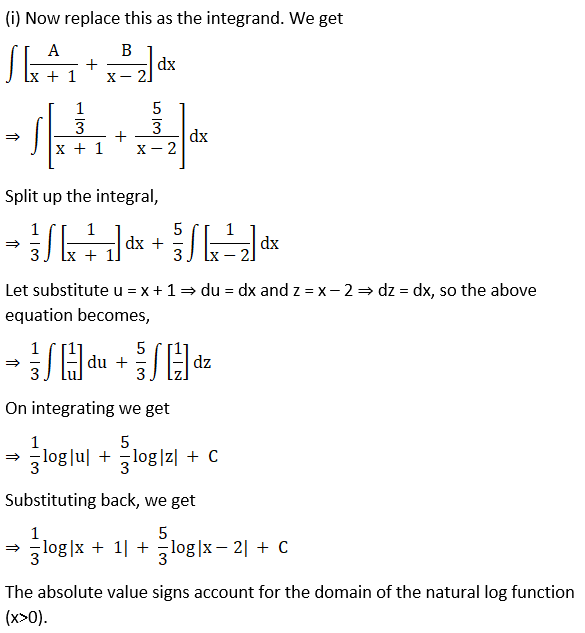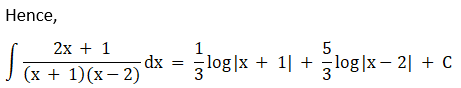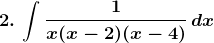Solution: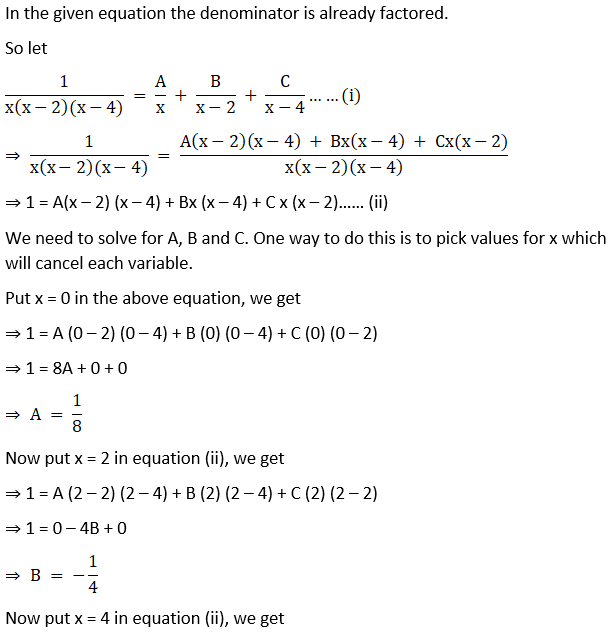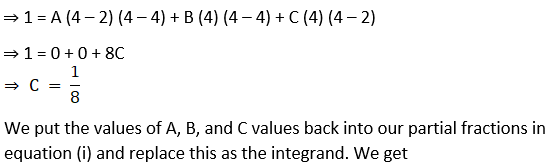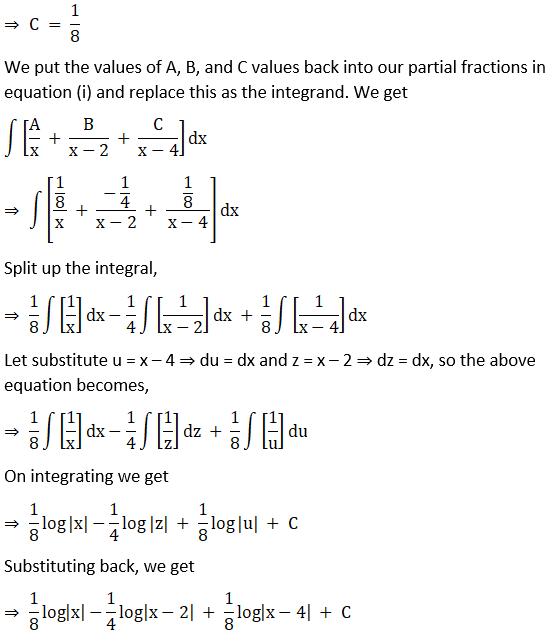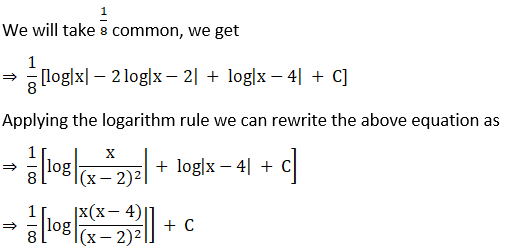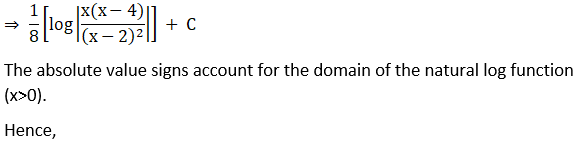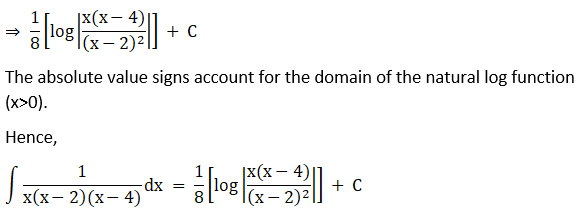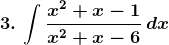Solution: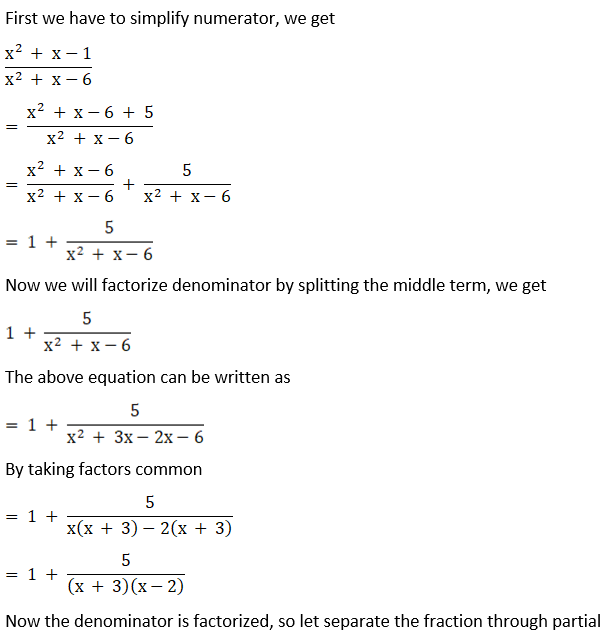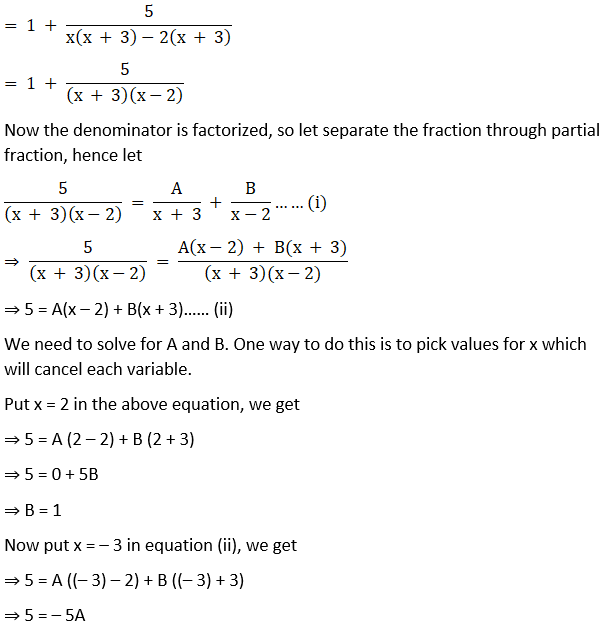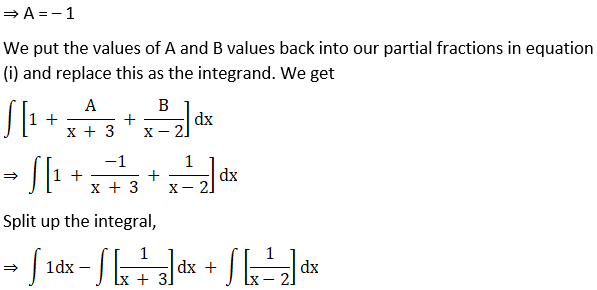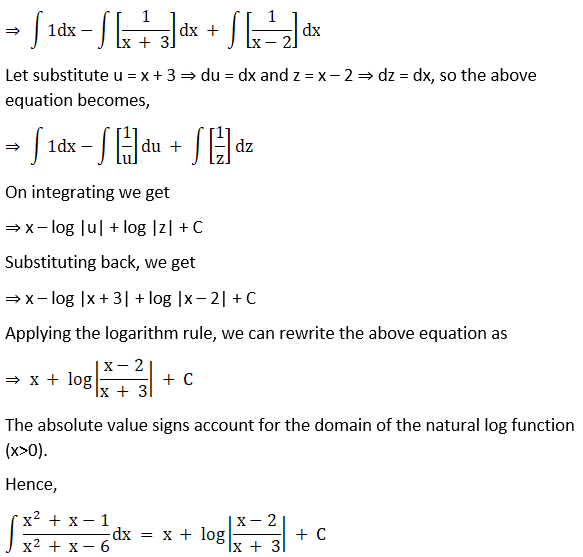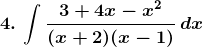Solution: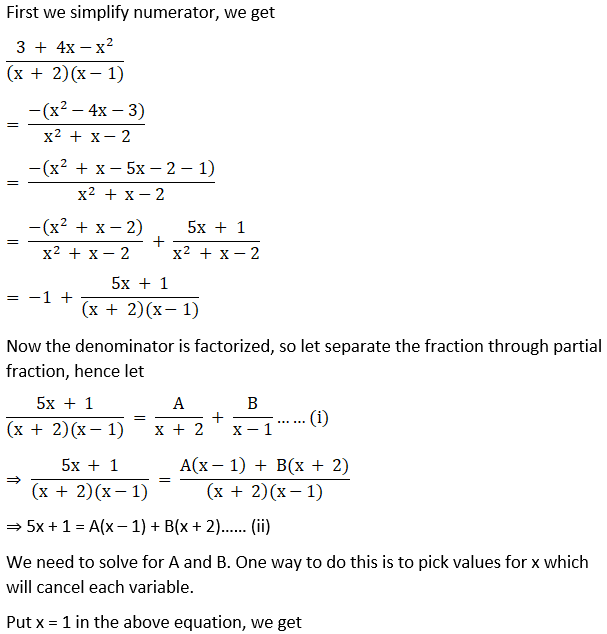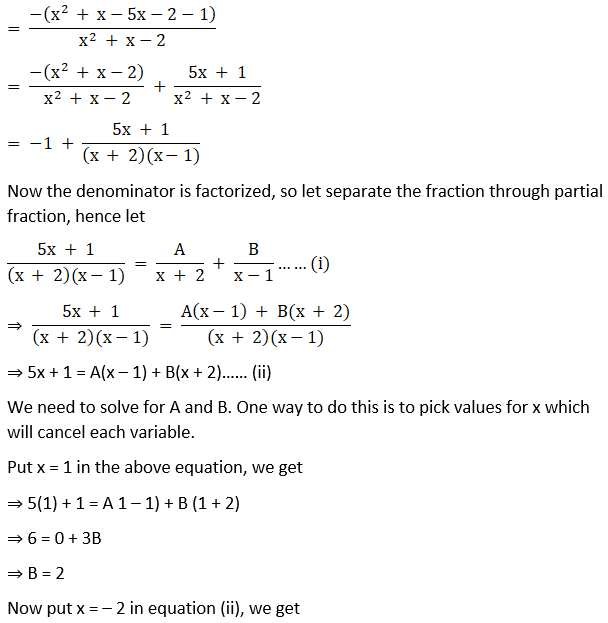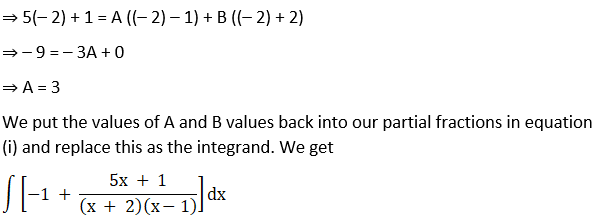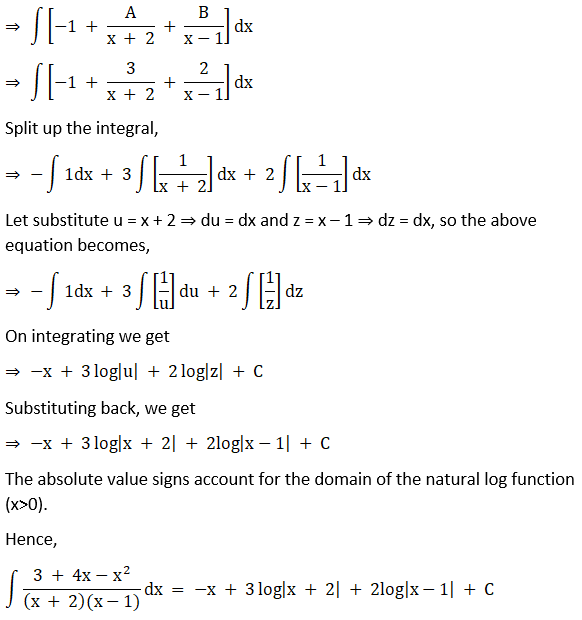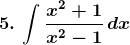Solution: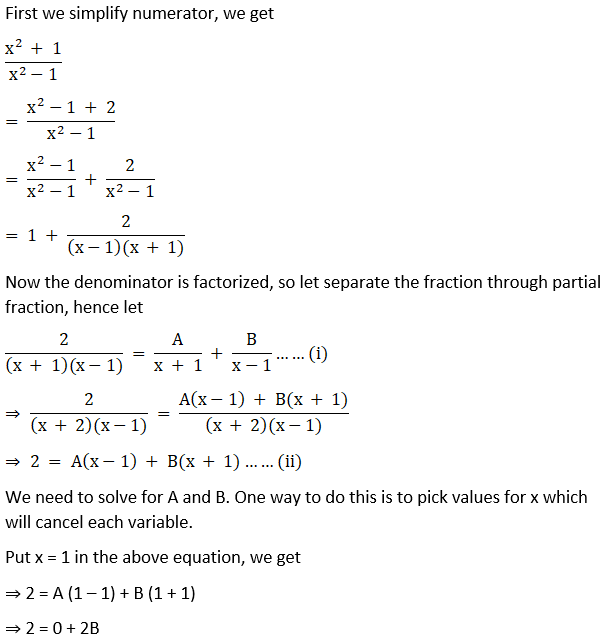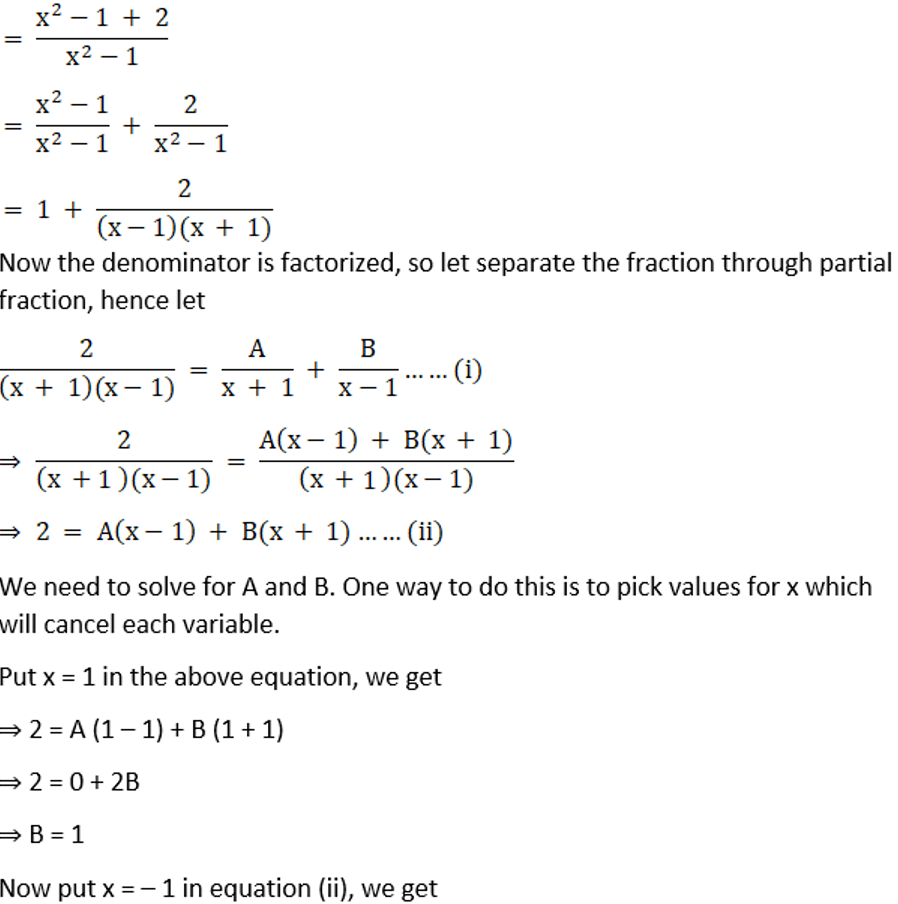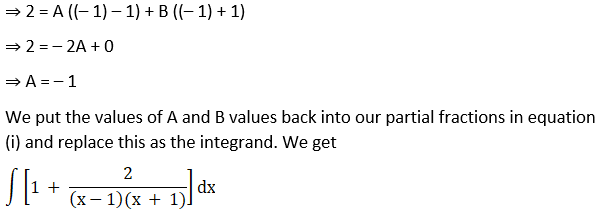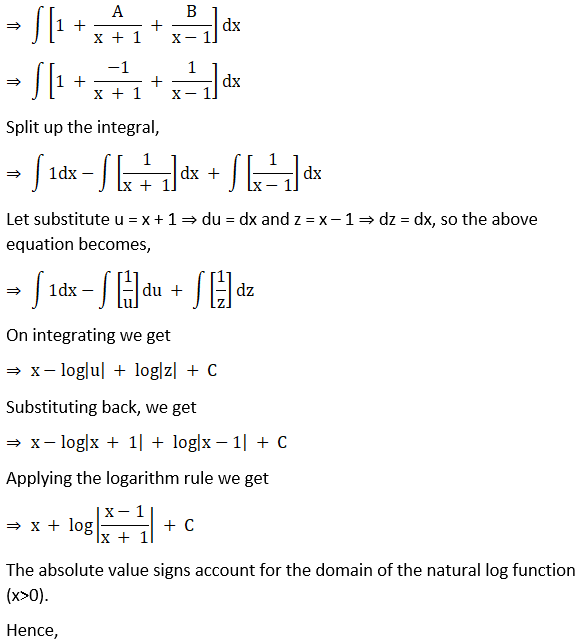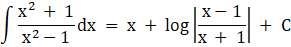### Access other exercises of RD Sharma Solutions For Class 12 Chapter 19 – Indefinite Integrals

Exercise 19.1 Solutions

Exercise 19.2 Solutions

Exercise 19.3 Solutions

Exercise 19.4 Solutions

Exercise 19.5 Solutions

Exercise 19.6 Solutions

Exercise 19.7 Solutions

Exercise 19.8 Solutions

Exercise 19.9 Solutions

Exercise 19.10 Solutions

Exercise 19.11 Solutions

Exercise 19.12 Solutions

Exercise 19.13 Solutions

Exercise 19.14 Solutions

Exercise 19.15 Solutions

Exercise 19.16 Solutions

Exercise 19.17 Solutions

Exercise 19.18 Solutions

Exercise 19.19 Solutions

Exercise 19.20 Solutions

Exercise 19.21 Solutions

Exercise 19.22 Solutions

Exercise 19.23 Solutions

Exercise 19.24 Solutions

Exercise 19.25 Solutions

Exercise 19.26 Solutions

Exercise 19.27 Solutions

Exercise 19.28 Solutions

Exercise 19.29 Solutions

Exercise 19.31 Solutions

Exercise 19.32 Solutions